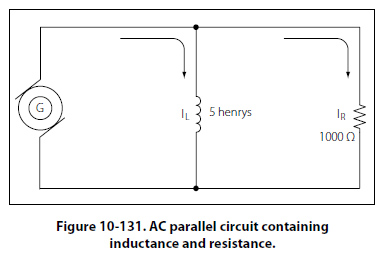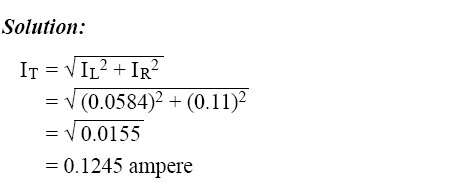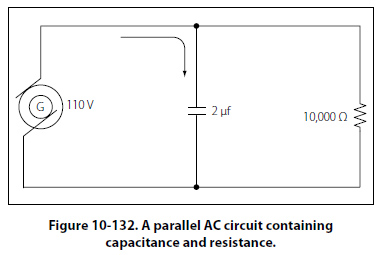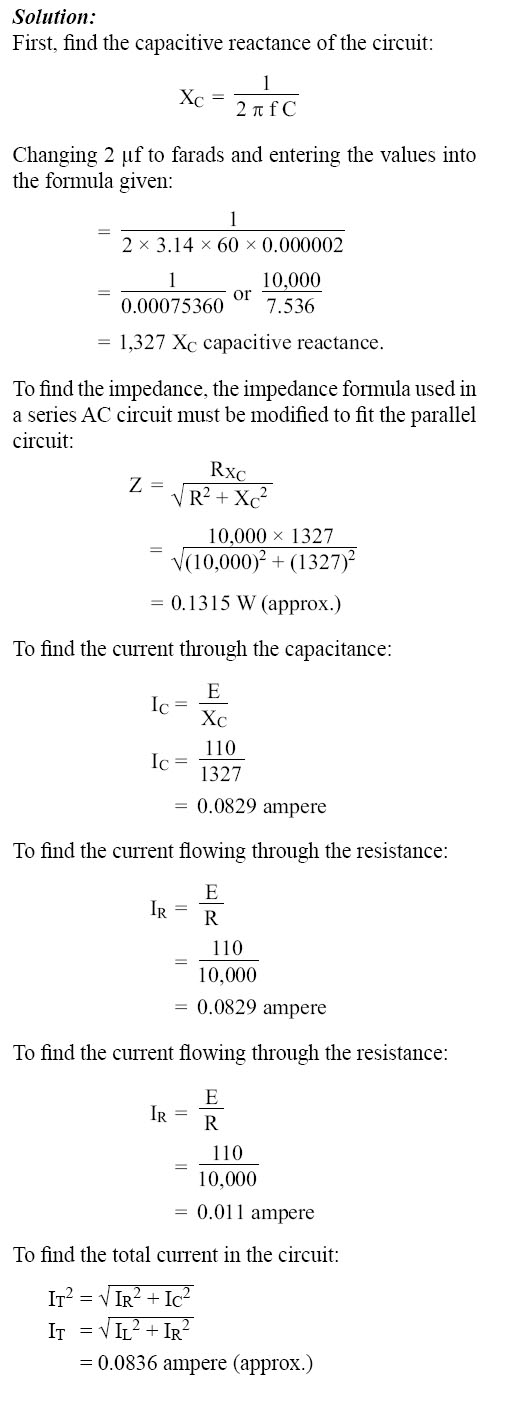Parallel AC Circuits The methods used in solving parallel AC circuit problems are basically the same as those used for series AC circuits. Out of phase voltages and currents can be added by using the law of right triangles. However, in solving circuit problems, the currents through the branches are added since the voltage drops across the various branches are the same and are equal to the applied voltage.In Figure 10-131, a parallel AC circuit containing an inductance and a resistance is shown schematically. The current flowing through the inductance, IL, is 0.0584 ampere, and the current flowing through the resistance is 0.11 ampere. What is the total current in the circuit?Since inductive reactance causes voltage to lead the current, the total current, which contains a component of inductive current, lags the applied voltage. If the current and voltages are plotted, the angle between the two, called the phase angle, illustrates the amount the current lags the voltage. In Figure 10-132, a 110-volt generator is connected to a load consisting of a 2 µf capacitance and a 10,000- ohm resistance in parallel. What is the value of the impedance and total current flow?©AvStop Online Magazine                                                                                                                                                      Contact Us              Return To Books